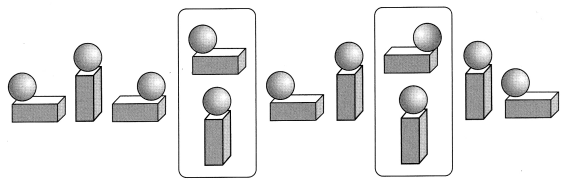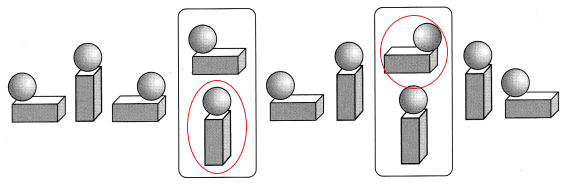# Math in Focus Grade 2 Chapter 19 Practice 3 Answer Key Making Patterns

This handy Math in Focus Grade 2 Workbook Answer Key Chapter 19 Practice 3 Making Patterns detailed solutions for the textbook questions.

## Math in Focus Grade 2 Chapter 19 Practice 3 Answer Key Making Patterns

Look at the pattern. Draw what comes next.

Example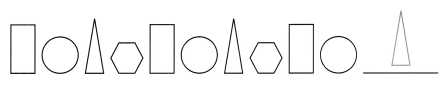Question 1.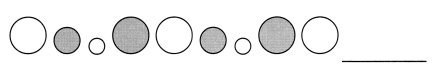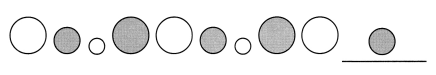Explanation:
Shape pattern occur when a group of shapes are repeated again and again
Circles of different sizes and colors are made as a pattern
I drew a small size colored circle to complete the pattern.

Question 2.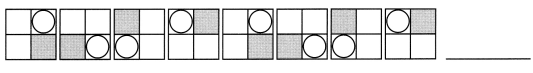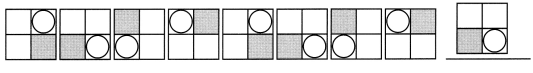Question 3.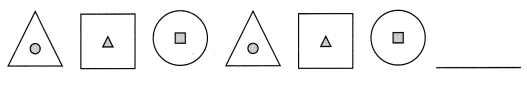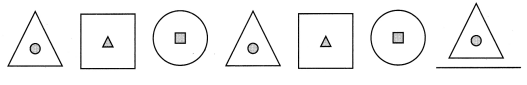Explanation:
Shape pattern occur when a group of shapes are repeated again and again
The shape pattern has a triangle, square and a circle
So, i drew a triangle to complete the pattern.

Circle the correct shapes or figures made of shapes to complete the pattern.

Example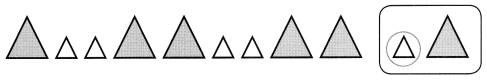Question 4.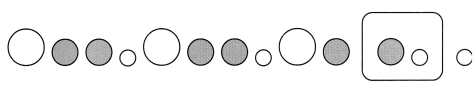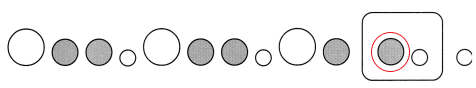Explanation:
Shape pattern occur when a group of shapes are repeated again and again
Circles of different sizes and colors are made as a pattern
I drew a small size colored circle to complete the pattern.

Question 5.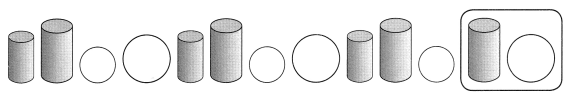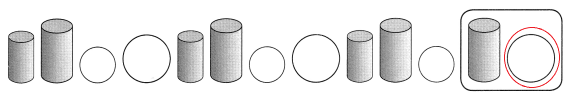Explanation:
Shape pattern occur when a group of shapes are repeated again and again
Circles of different sizes and cylinders are made as a pattern
I drew a big size  circle to complete the pattern.

Question 6.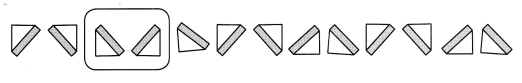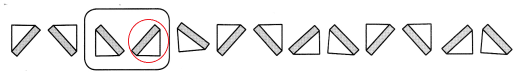Explanation:
Shape pattern occur when a group of shapes are repeated again and again
Triangular pieces of different directions are made as a pattern
I circled the shape that is correct among the 2 to complete the pattern.

Question 7.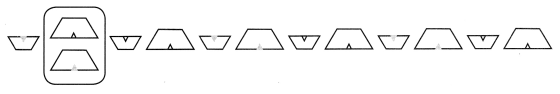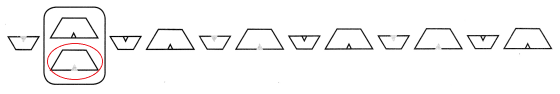Explanation:
Shape pattern occur when a group of shapes are repeated again and again
I circled the shape that is correct among the 2 to complete the pattern.

Circle the correct shapes or figures made of shapes to complete the pattern.

Question 8.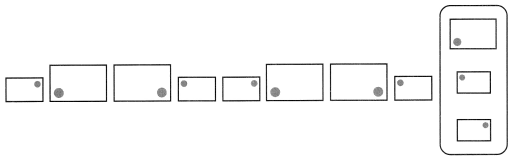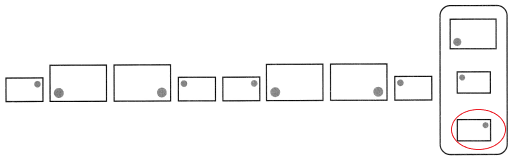Explanation:
Shape pattern occur when a group of shapes are repeated again and again
I circled the shape that is correct among the 3 to complete the pattern.

Question 9.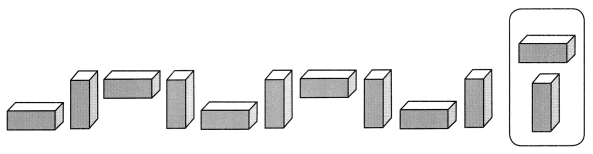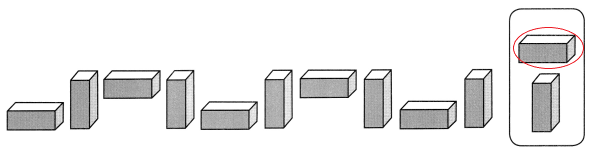Explanation:
Shape pattern occur when a group of shapes are repeated again and again
I circled the shape that is correct among the 2 to complete the pattern.

Question 10.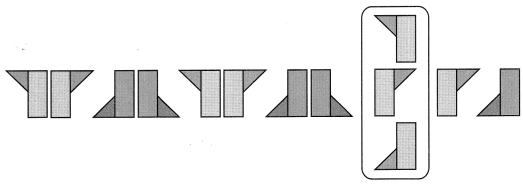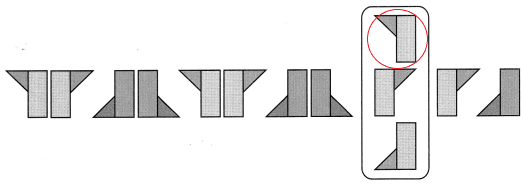Explanation:
Shape pattern occur when a group of shapes are repeated again and again
I circled the shape that is correct among the 3 to complete the pattern.

Circle the correct shapes or figures made of shapes to complete the pattern.

Question 11.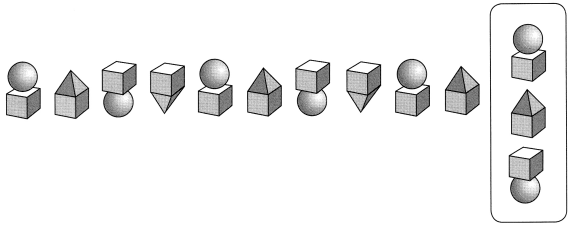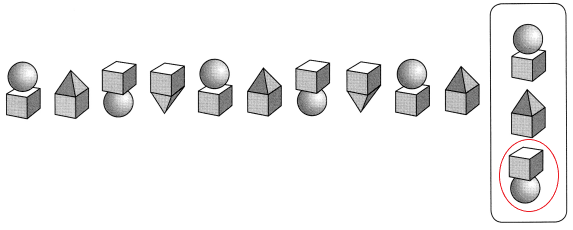Explanation:
Shape pattern occur when a group of shapes are repeated again and again
I circled the shape that is correct among the 3 to complete the pattern.

Question 12.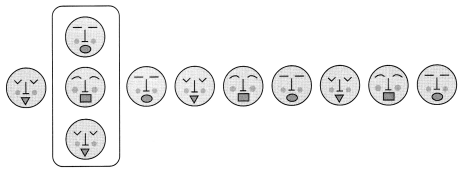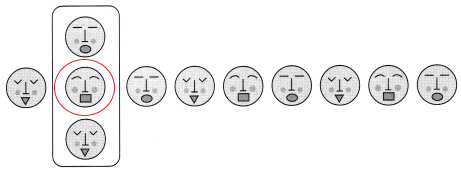Explanation:
Shape pattern occur when a group of shapes are repeated again and again
I circled the shape that is correct among the 3 to complete the pattern.

Question 13.